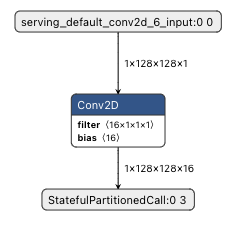# The SRAM allocation with Vela compiler

I have two questions about vela compiler compile my tensorflow ilte model (ethos u55 with 128MACs)

I try to use vela compiler compile two model  one have kernel with 16 channels and one have 17 channels

the vela report the allocation peak tensor size(bytes) , total sram used (KiB) , NPU cycles(cycles/batch) , batch inference times(ms)

and i observe the following situation :

Tensor refers to the feature map size, our input is 128x128=16384, and our model channel number is set to 16, so the second layer Tensor size is 128x128x16=262,144, and there is an overlap of the part is the size of the SRAM allocation, so Allocation Peak Tensor Size = 262144x2 = 524288 Bytes , Total SRAM used = 512 KiB. The final NPU cycle is 2795457cycle, Batch Inference time = 5.59 ms at clokc=500MHz.

If other conditions remain unchanged and the model with 17 channels is changed, after running, we can see from Fig. 4.7 that the second layer Tensor size was originally thought to be 128x128x17=278528, but it is actually 524288, which means we can know whether the number of channels of the model is a multiple of 16 or not, which has a serious impact on the allocation of memory resources. allocation Peak Tensor Size = 524288 x 2 =1064960 Bytes, Total SRAM used = 1040 KiB, Final NPU cycle is 5228677cycle, Batch Inference time=10.47 ms at clokc=500MHz.

1. It seems vela compiler allocate tensor sram size depends on the NN-model's max channel and with 16 channels as a unit?

2.Why does the total SRAM used seriously affect the NPU cycles, as the above report points out that the SRAM usage of the channel 17 model is twice as much as that of the channel 16 model (the same parameter of the vela compiler figure-shared SRAM mode), then the NPU cycles are almost the same as the amount of SRAM used? Is this DMA related?

thank you!

• Hi,

I've got some answers from a Vela expert:

1. Vela tries to allocate most feature maps with a channel depth that is a multiple of 16 bytes (see NHCWB16 memory format, TRM section 3.1.1). It does this because it provides the most efficient memory accesses.
2. The Ethos-U uses block based processing with performance that is defined in the Ethos-U55 Technical Reference Manual (TRM) - https://documentation-service.arm.com/static/6305faeffc87ea23a5e0aa18 sections 4.8.3 and 4.8.4.
There is mention of figure 4.7, but we don't have that, does that give the extra info we need? Are there pictures/tables/links that didn't make it into the message?
• Thank you for getting in touch.

Good question - let me try and explain what is going on. I define a model like what you describe- input 128x128 in greyscale, CONV_2D with 16 channels, see picture of the model below.I use int8 activations on the inputs. Assuming the weights of the NN are stored in the SRAM, you will compile the model with the following command:

`\$ vela conv_16channels.tflite --accelerator-config=ethos-u55-128 --config <path to your .ini> --memory-mode=Sram_Only --system-config=Ethos_U55_High_End_Embedded`

How much SRAM do you need to store the peak tensor during the inference? You need to have enough space to hold the Input Feature Map + the Outpute Feature Map, in other words 128*128 + 128*128*16 = 278528 bytes. Vela reports Total SRAM Used of 272KB which is exactly 278528 bytes.

Now, assume instead of placing the model in SRAM, you place the model in the Flash. This means that you have to compile for Shared_Sram memory mode. This time Vela reports Total SRAM Used of 272.19KB so we've got a slight increase in memory footprint. This is because Vela is buffering the weights of the NN from the Flash to the SRAM. In other words, Vela issues DMA commands that will move weights from Flash to SRAM so that when the NPU needs those weights, they are already available in the SRAM. If you compile with --verbose-all, you are going to see that there are 192 bytes of weights that are buffered into SRAM. And indeed 128*128 + 128*128*16 + 192 is equal to exactly 272.19KB.

If I change the number of channels of the model to 17, the calculation is exactly the same. For Sram_Only memory mode(weights in the SRAM), the Total SRAM Used is equal to 128*128*17+128*128= 294912 bytes = 288 KB and Vela reports Total SRAM of 288KB. For Shared_Sram memory mode(weights in the Flash), the total SRAM is equal to 288.22KB because of the buffering as explained in the previous case.

Hope that clarifies the logic behind the tensor allocation in Vela.

You also speak about the reported NPU Cycles and Batch inference time - please know these numbers are only rough estimates and won't match performance in silicon. Vela does not have cycle accurate model of the ethos-U hardware so it can't predict the exact behaviour of the hardware. To get accurate performance figures, you need to run your neural network on the Fixed Virtual Platform or on the MPS3 FPGA board. This project(review.mlplatform.org/.../ml-embedded-evaluation-kit) explains how you cando that.